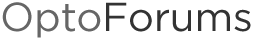# Programmatically Change your IP Settings

PAC Control includes a command to get your PAC’s address (Get Control Engine Address), but what if you need to change some IP Settings either locally or on another mem map device, without using PAC Manager?

Near the end of form 1465 (in the IP Settings–Read/Write section), you’ll find instructions on how to do this, but I always have to look up what “1s complement” means (bitnot)…

Anyway, it’s a 2-step process which does NOT require a store to flash, but DOES require a reboot to take effect. The instructions, direct from form 1465: IP Settings—Read/Write
If you are not using PAC Manager to change IP addressing for the Ethernet network interface(s) on the device, you can use this area of the memory map to do so. All changes made in this area require two steps (PAC Manager does these steps automatically):

1. Send the new address (8 bytes consisting of the address [4 bytes] plus the 1s complement of it
[4 bytes]). The 1s complement requirement is designed to avoid accidental changes.

2. Send a reset hardware command (see Status Area Write address F0380000, operation code
0x00000005) to cycle power and make the changes take effect.

Sample OptoScript code:

``````// Here are some sample address values (these might come from somewhere else, like groov or PAC Display)

// First write the IP address & subnet mask
ntMemMapData = bitnot nIPAddress; // 1s Complement

ntMemMapData = bitnot nSubnetMask; // 1s Complement

nResult = WriteNumTableToIoUnitMemMap(4, 0, self, 0xFFFFF050, ntMemMapData);

// Now write the default gateway address & DNS
ntMemMapData = nGateway;
ntMemMapData = bitnot nGateway; // 1s Complement

ntMemMapData = nDNS;
ntMemMapData = bitnot nDNS; // 1s Complement

nResult = WriteNumTableToIoUnitMemMap(4, 0, self, 0xFFFFF068, ntMemMapData);

// Last step, send hardware reset (USE WITH CAUTION IF WRITING TO SELF)
nResult = WriteNumToIoUnitMemMap( self, 0xF0380000, 5 );

``````

Note that this example was for the Secondary IP settings, for Primary settings (as documented in form 1465) the 0xFFFFF050 & 0xFFFFF068 addresses above would need to be: 0xFFFFF008 & 0xFFFFF018 instead.

Note: As you can probably guess, SoftPAC does NOT include this part of the memory map. But you could run this code on a SoftPAC controller, you’d just make sure that the I/O Unit I called “self” in the example above would have to correspond to a non-SoftPAC.

-OptoMary

Thank you very much for this post! This was a big help in figuring out how to set the IP address using the OptoMPP Communication Toolkit as well.

I have the Opto 22 SNAP Ethernet I/O Command Line Tool C++ program (eiocl) installed on a Linux system that is connected to a SNAP-PAC-R1 controller. I was able to use this program to write a new IP address to the R1 controller. Here’s how it works:

First, determine your new IP address. For this example, I’ll use 33.44.128.11. Then figure out the hexadecimal byte values of each of the four numbers in the IP address. For this example: 33 = 0x21, 44 = 0x2C, 128 = 0x80, and 11 = 0x0B

Then, invert the bits in each of these four hexadecimal byte values. Here’s how to invert the bits:
0=F, 1=E, 2=D, 3=C, 4=B, 5=A, 6=9, 7=8, 8=7, 9=6, A=5 B=4, C=3, D=2, E=1, and F=0.

So, in this example: 0x21 = 0xDE, 0x2C = 0xD3, 0x80 = 0x7F, 0x0B = 0xF4.

Then, this is the command-line for the eiocl program to write the Secondary IP address. After
the “w b 8” which indicates write 8 bytes, put the four hexadecimal byte values for the new IP address
followed by the four hexadecimal byte values of the inverted IP address:
\$INSTALL_PATH/snapenetdtk_R20a/Examples/C++/Linux/eiocl/eiocl \$TARGET_IP 2001 10 FFFFF050 w b 8 21 2C 80 0B DE D3 7F F4

This is the command-line for the eiocl program to read the Secondary IP address that was just set:
\$INSTALL_PATH/snapenetdtk_R20a/Examples/C++/Linux/eiocl/eiocl \$TARGET_IP 2001 10 FFFFF050 r ip

This is the command-line for the eiocl program to power-reset the R1 controller:
\$INSTALL_PATH/snapenetdtk_R20a/Examples/C++/Linux/eiocl/eiocl \$TARGET_IP 2001 10 F0380000 w b 4 0 0 0 5